We live in the time domain. This is where we measure all digital performance. But sometimes, we can get to an answer faster by taking a detour through the frequency domain. With six principles, we can understand how an oscilloscope transforms time domain measurements into a frequency domain view. All six principles are applied “under the hood” by oscilloscopes with a Fast Fourier Transform (FFT) function.

### 1. The Spectrum Is a Combination of Sine Wave Components

In the frequency domain, the only waveforms we are allowed to consider are sine waves. There are other special waveforms, combinations of which can describe any time-domain waveform, such as Legendre polynomials, Hermite polynomials, or even wavelets, but the reason we single out sine waves for a frequency domain description is that sine waves are solutions to second order, linear, and differential equations — the equations found so often in electrical circuits involving resistor, capacitor, and inductor elements. This means signals that arise or have interacted with RLC circuits are described more simply when using combinations of sine waves than any other function because sine waves naturally occur.

A sine wave measured by an oscilloscope with one million voltage-time [V(t)] data points in the acquisition buffer (Figure 1) is described by only three numbers in the frequency domain: a frequency value, an amplitude value, and a phase value. This is a dramatic simplification of the original complex waveform.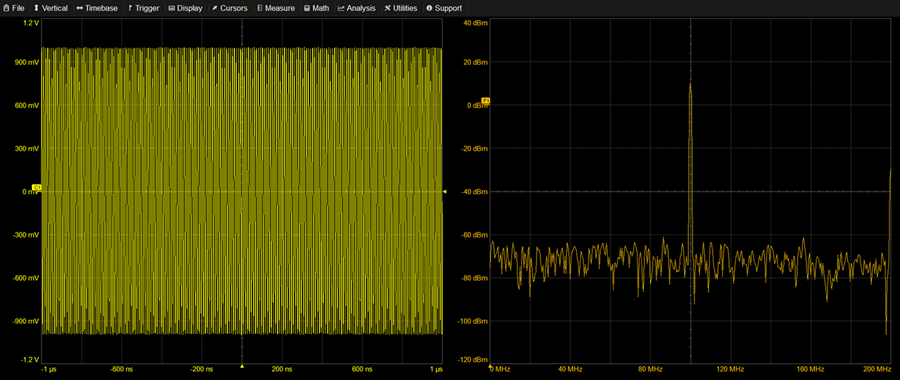Figure 1. A 100 MHz sine wave in the time domain and its spectrum in the frequency domain showing the one peak at 100 MHz.

### 2. Appending A Waveform to Itself Infinitely Creates a Periodic Waveform

When we take a waveform in the time domain and transform it into the frequency domain, we end up with a collection of sine waves, each with a frequency value, an amplitude, and a phase. In the time domain, we describe the measurements as an acquisition buffer with a total acquisition time, T, and a time interval between samples, T. When we describe the same waveform in the frequency domain, we refer to the collection of all the sine wave components — each with a frequency, amplitude, and phase — as the spectrum.

Unfortunately, we can only use the Discrete Fourier Transform (DFT) on a V(t) waveform that is periodic. If it is not periodic, we must artificially make it periodic. The trick we use to turn any arbitrary acquisition buffer of measured data into a periodic waveform is to take the acquisition buffer of total time, T, and repeat it forever in the past and forever in the future.

When we have this artificially repetitive waveform, we can apply the power of the DFT to mathematically calculate each frequency component in the spectrum. Here are the equations used to calculate the amplitude and phase of each frequency component: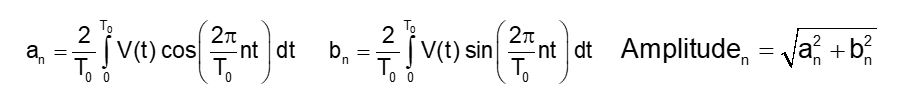These integrals create certain features in the spectrum.

### 3. Only Discrete Frequencies Appear in a Spectrum; the Lowest Is the Fundamental

In the calculated spectrum, only discrete frequency values appear. The lowest frequency component is called the fundamental. It is the lowest frequency sine wave we can fit into the acquisition buffer time. The period, P, of this lowest frequency sine wave, is the total acquisition time, T.

Since P equals T, the fundamental frequency is: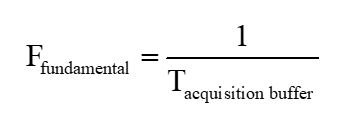Each frequency component in the spectrum has a frequency that is only an integer multiple of the fundamental: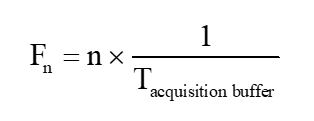Multiples of the fundamental are the only frequency components we will see in the spectrum. This means, the frequency spacing between each frequency component, or the resolution, is the fundamental frequency. If we want a higher resolution to distinguish closer spaced frequency features in the spectrum, we need to use a longer acquisition time in the oscilloscope.

### 4. The Highest Frequency Is the Nyquist, or One-Half the Sample Rate

The highest frequency component in the spectrum is related to the time interval between the sampled points in the buffer. At a minimum, we need two measured V(t) points in one period to calculate a value for the amplitude and phase of that frequency component. This means the period of the highest frequency sine wave we can calculate is twice the time interval, or Pmax = 2 x T.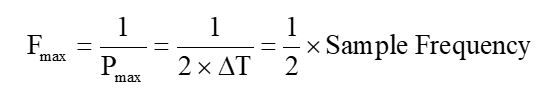The highest frequency component in the spectrum is also referred to as the Nyquist frequency. Since the sample rate for taking data is 1/T, the Nyquist frequency, the highest frequency for which we can calculate a sine wave component is one-half the sample rate. If the sample rate is 10 GS/s, the Nyquist frequency is 5 GHz.

The average value of an ideal sine wave is always 0. This means that when we use a collection of sine waves to describe a real waveform, the average value of the recreated time-domain waveform is always 0. But real waveforms have an average value, or DC offset. To account for this, we store the DC component in the 0 Hz frequency component, which is 0 x the fundamental. In most oscilloscopes, you can suppress plotting the 0th frequency component to zoom in on the scale of the display.

### 5. The FFT Speeds Calculation by First Truncating the Buffer to 2^n Sample Points

One million data points would involve about one trillion DFT calculations to create one spectrum. This may take longer to calculate than is convenient. To get around this problem, we use the much faster version of the DFT called FFT. It calculates the same integrals as the DFT, but it applies matrix math to perform the calculations using a total number of points that is a power of 2. If there are one million points in the buffer, the highest number of points that could be included in the FFT calculation would be 2^19 = 524,288 points. We throw out almost half the measured data to gain incredibly fast computation time.

The first step in performing an FFT is to define the region of the acquisition buffer that contains the largest number of 2^n points that will be computed. Most oscilloscopes allow you to pick either the central region of the time-domain screen or count from the left edge. Figure 2 shows the region that will be included in the FFT calculation, between the dashed lines.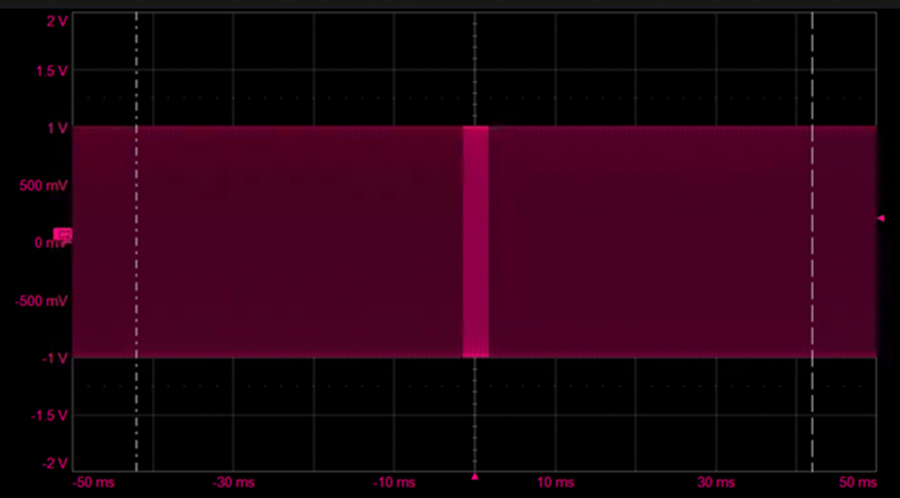Figure 2. Between the vertical dashed lines is the region of the acquisition buffer that contains the 2^n points which will be used in the FFT.

When the acquisition buffer time is 1 µs, and we have one million points, we expect the fundamental to be 1 MHz. In the spectrum, the FFT acquisition buffer is smaller than this, which means the actual resolution is slightly larger than 1 MHz. But these estimates are still a good value to use when thinking about the features of the spectrum.

### 6. Windowing Functions Prevent Spectral Leakage Due to the Truncation

To create a periodic waveform, we took the acquisition buffer and repeated it indefinitely. When using the FFT function, we further truncated the acquisition buffer and repeat the truncated buffer indefinitely. This means that at the boundaries of each appended acquisition buffer, there may be a discontinuity in the waveform corresponding to the end of one buffer and the beginning of the next one (Figure 3).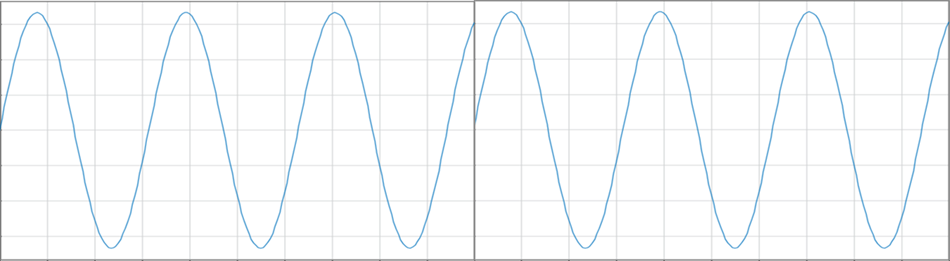Figure 3. Example of a sine wave signal which does not have an integral number of cycles in the acquisition buffer. When appended to each other, there is a discontinuity in the waveform at each edge of the buffer, courtesy of National Instruments.

Normally, the spectrum of a sine wave that has an integral number of cycles in each buffer will have a single frequency spike at its peak frequency. However, if the sine wave is artificially cut off due to the truncated acquisition buffer, the infinitely long waveform will now have a discontinuity that will force some of the frequency components from the peak frequency into adjacent frequency components, which can result in a distortion of the narrow peak.

This effect is called spectral leakage. It is an artifact of the discontinuity at the boundaries of the buffers due to the first voltage value not being the same as the last voltage value. The way to reduce this artifact is to artificially reduce the discontinuity by multiplying the entire acquisition buffer by a window function. This gradually forces the voltage value at the ends of the acquisition buffer to be 0, guaranteeing that the end of one buffer is continuous with the beginning of the next buffer.

There are a number of windowing functions commonly used. They differ in how much spectral leakage they allow and the resulting resolution. Unless you have a strong compelling reason, we recommend that you always use either the von Hann (sometimes called Hanning) or the Blackman-Harris function.

### Analyzing Time Domain Waveforms in the Frequency Domain

The value of spectral analysis is being able to identify the spectral “fingerprint” of repetitive signals with frequencies that fit within the range between the fundamental (1/acquisition buffer) and the Nyquist (½ x sample rate). Each time the oscilloscope measures a new acquisition buffer of time-domain voltages, the newly calculated spectral response is displayed. As periodic signals in the source change, their spectral fingerprint changes.

Any application where it might be helpful to identify periodic signals of a given frequency is a great candidate for real-time spectral analysis. The most common application is searching for the sources of interference on important signals. Figure 4 shows the spectral response of the output of a switch-mode power supply (SMPS) as its load was changed. The peaks at about 50 kHz, the switching frequency changes over time as the load changes, as shown by a spectrogram in the upper part of the figure. When you observe the noise of an amplifier or the jitter of an oscillator within these frequency components, you have an idea of a possible root cause.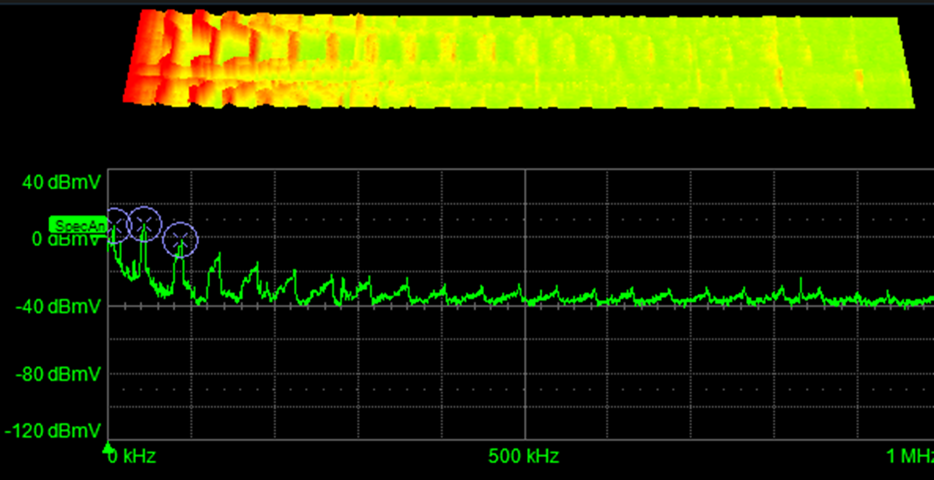Figure 4. An example of the real-time spectrum of a SMPS output while the output load is changing showing the time variation of the peak frequencies.

When looking at the spectrum of RF interference, we can often pick up specific communications signals. Figure 5 shows the real-time spectrum of the power rail in a USB-powered device measured using a 10x probe with a large tip loop. The spectral fingerprint shows pick up in the FM radio band from 87 MHz to 108 MHz.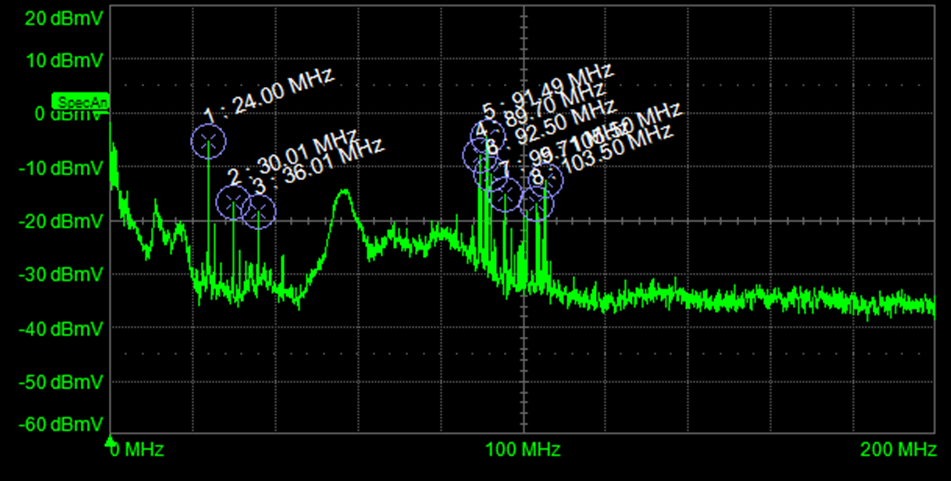Figure 5. Measured real-time spectrum of the noise from a USB power rail showing large components in the FM radio band from pick up in the probe.